# Functional Reactive Programming without Black Magic (UIKonf 2015)

May. 19, 2015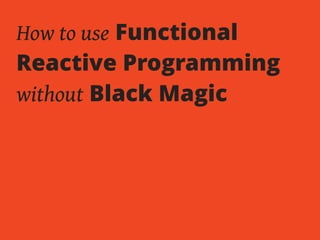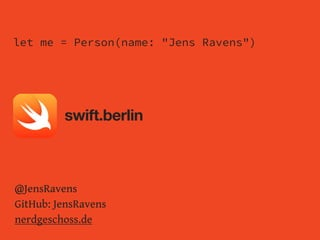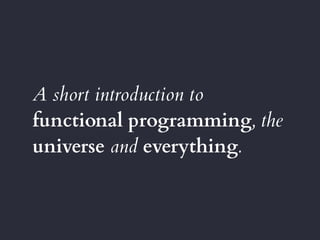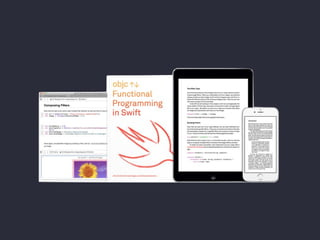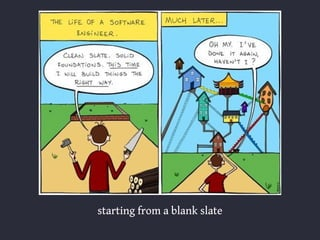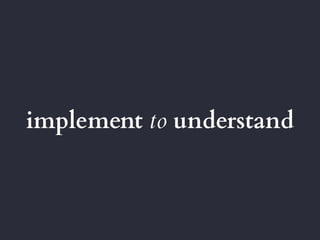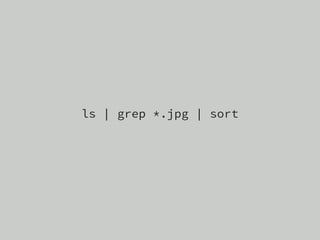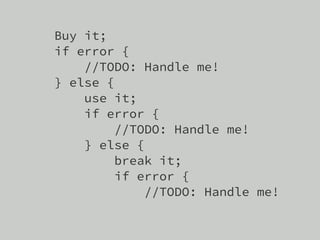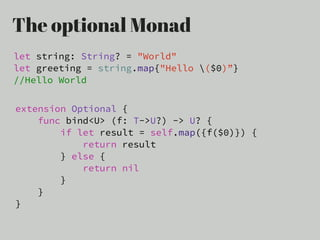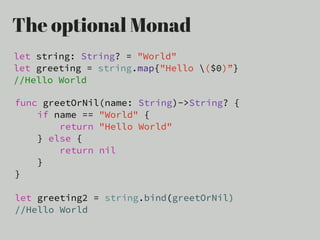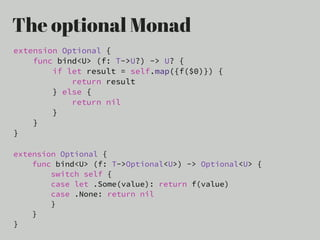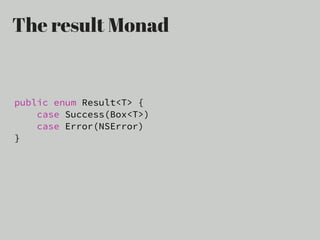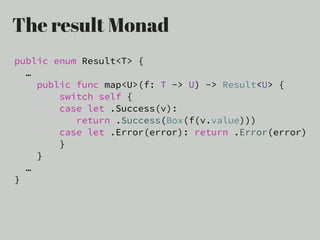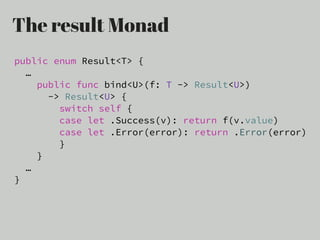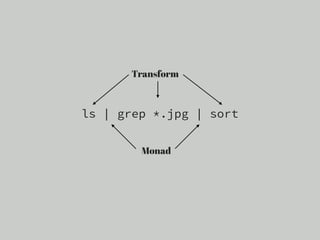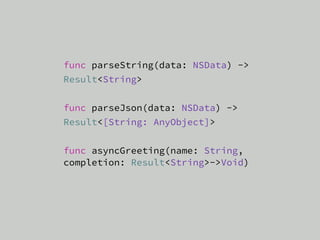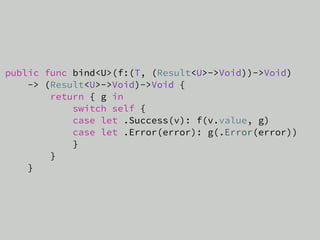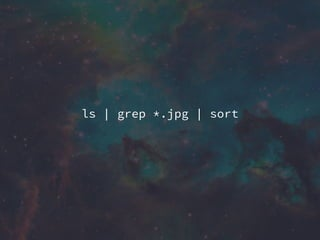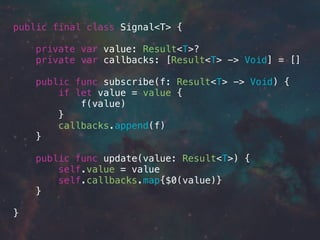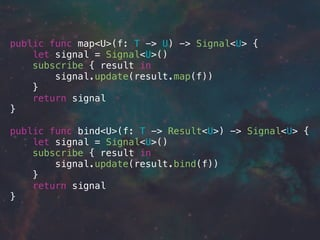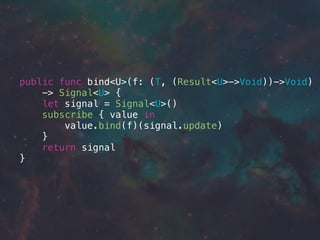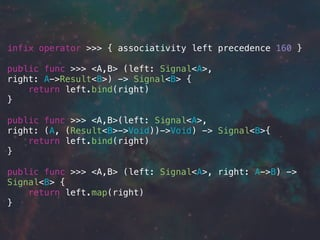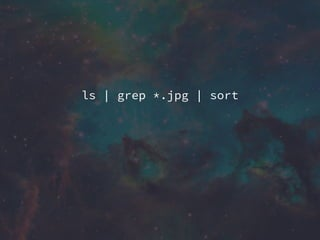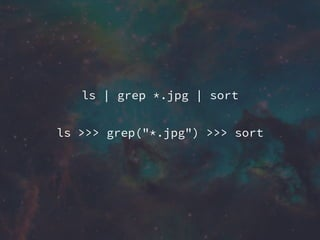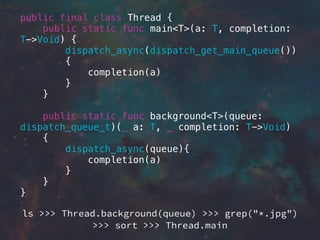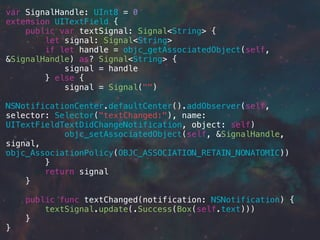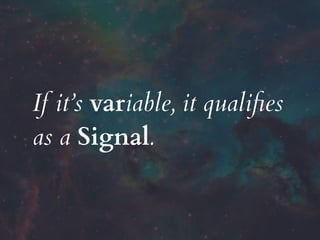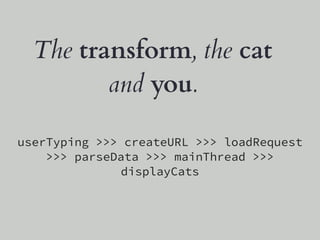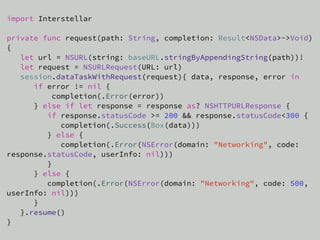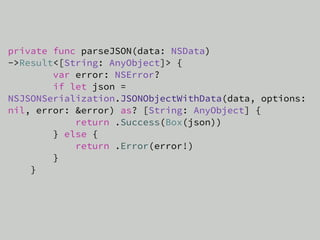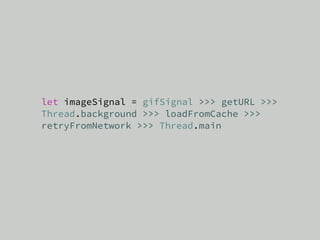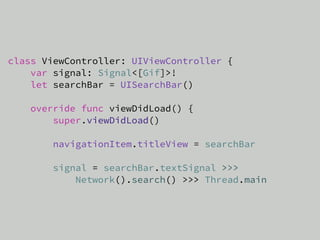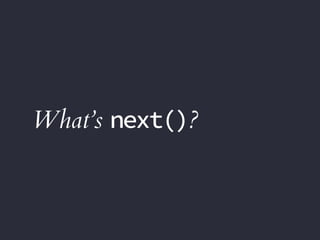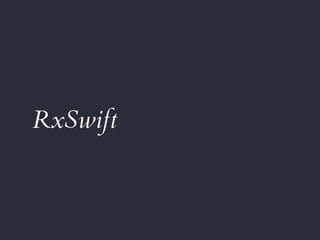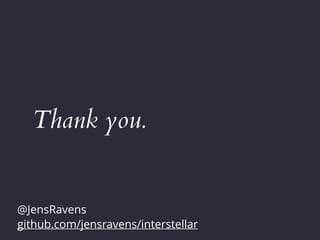1 of 55

### Functional Reactive Programming without Black Magic (UIKonf 2015)

• 1. How to use Functional Reactive Programming without Black Magic
• 2. let me = Person(name: "Jens Ravens") @JensRavens GitHub: JensRavens nerdgeschoss.de swift.berlin
• 3. A short introduction to functional programming, the universe and everything.
• 4. In the beginning McIlroy created the unix pipe. And he saw it was good. ls | grep *.jpg | sort
• 5. Application Architecture
• 7. starting from a blank slate
• 8. implement to understand
• 9. And I promise not to use the scary M-word. me, 12 weeks ago.
• 11. –Saunders Mac Lane All told, a monad is just a monoid in the category of endofunctors. “
• 12. Monads are just Applicative Functors “
• 13. ls | grep *.jpg | sort
• 14. Buy it, use it, break it, fix it, Trash it, change it, mail - upgrade it. – Daft Punk, Technologic
• 15. Buy it; if error { //TODO: Handle me! } else { use it; if error { //TODO: Handle me! } else { break it; if error { //TODO: Handle me!
• 16. ls | grep *.jpg | sort
• 17. Monad something that defines map and bind
• 18. The optional Monad let string: String? = "World" let greeting = string.map{"Hello (\$0)”} //Hello World extension Optional { func bind<U> (f: T->U?) -> U? { if let result = self.map({f(\$0)}) { return result } else { return nil } } }
• 19. The optional Monad let string: String? = "World" let greeting = string.map{"Hello (\$0)”} //Hello World func greetOrNil(name: String)->String? { if name == "World" { return "Hello World" } else { return nil } } let greeting2 = string.bind(greetOrNil) //Hello World
• 20. The optional Monad extension Optional { func bind<U> (f: T->U?) -> U? { if let result = self.map({f(\$0)}) { return result } else { return nil } } } extension Optional { func bind<U> (f: T->Optional<U>) -> Optional<U> { switch self { case let .Some(value): return f(value) case .None: return nil } } }
• 21. The result Monad public enum Result<T> { case Success(Box<T>) case Error(NSError) }
• 22. The result Monad public enum Result<T> { … public func map<U>(f: T -> U) -> Result<U> { switch self { case let .Success(v): return .Success(Box(f(v.value))) case let .Error(error): return .Error(error) } } … }
• 23. The result Monad public enum Result<T> { … public func bind<U>(f: T -> Result<U>) -> Result<U> { switch self { case let .Success(v): return f(v.value) case let .Error(error): return .Error(error) } } … }
• 24. ls | grep *.jpg | sort Monad Transform
• 25. func parseString(data: NSData) -> Result<String> func parseJson(data: NSData) -> Result<[String: AnyObject]> func asyncGreeting(name: String, completion: Result<String>->Void)
• 26. public func bind<U>(f:(T, (Result<U>->Void))->Void) -> (Result<U>->Void)->Void { return { g in switch self { case let .Success(v): f(v.value, g) case let .Error(error): g(.Error(error)) } } }
• 27. Interstellar
• 28. ls | grep *.jpg | sort
• 29. public final class Signal<T> { private var value: Result<T>? private var callbacks: [Result<T> -> Void] = [] public func subscribe(f: Result<T> -> Void) { if let value = value { f(value) } callbacks.append(f) } public func update(value: Result<T>) { self.value = value self.callbacks.map{\$0(value)} } }
• 30. public func map<U>(f: T -> U) -> Signal<U> { let signal = Signal<U>() subscribe { result in signal.update(result.map(f)) } return signal } public func bind<U>(f: T -> Result<U>) -> Signal<U> { let signal = Signal<U>() subscribe { result in signal.update(result.bind(f)) } return signal }
• 31. public func bind<U>(f: (T, (Result<U>->Void))->Void) -> Signal<U> { let signal = Signal<U>() subscribe { value in value.bind(f)(signal.update) } return signal }
• 32. pushing instead of pulling
• 33. the rise of custom operators
• 34. infix operator >>> { associativity left precedence 160 } public func >>> <A,B> (left: Signal<A>, right: A->Result<B>) -> Signal<B> { return left.bind(right) } public func >>> <A,B>(left: Signal<A>, right: (A, (Result<B>->Void))->Void) -> Signal<B>{ return left.bind(right) } public func >>> <A,B> (left: Signal<A>, right: A->B) -> Signal<B> { return left.map(right) }
• 35. ls | grep *.jpg | sort
• 36. ls | grep *.jpg | sort ls >>> grep("*.jpg") >>> sort
• 38. public final class Thread { public static func main<T>(a: T, completion: T->Void) { dispatch_async(dispatch_get_main_queue()) { completion(a) } } public static func background<T>(queue: dispatch_queue_t)(_ a: T, _ completion: T->Void) { dispatch_async(queue){ completion(a) } } } ls >>> Thread.background(queue) >>> grep("*.jpg") >>> sort >>> Thread.main
• 39. Extending UIKit to support Signals.
• 40. var SignalHandle: UInt8 = 0 extension UITextField { public var textSignal: Signal<String> { let signal: Signal<String> if let handle = objc_getAssociatedObject(self, &SignalHandle) as? Signal<String> { signal = handle } else { signal = Signal("") NSNotificationCenter.defaultCenter().addObserver(self, selector: Selector("textChanged:"), name: UITextFieldTextDidChangeNotification, object: self) objc_setAssociatedObject(self, &SignalHandle, signal, objc_AssociationPolicy(OBJC_ASSOCIATION_RETAIN_NONATOMIC)) } return signal } public func textChanged(notification: NSNotification) { textSignal.update(.Success(Box(self.text))) } }
• 41. If it’s variable, it qualiﬁes as a Signal.
• 42. ReactiveKitten It’s about gifs. And cats. And gifs of cats.
• 43. userTyping >>> createURL >>> loadRequest >>> parseData >>> mainThread >>> displayCats The transform, the cat and you.
• 44. import Interstellar private func request(path: String, completion: Result<NSData>->Void) { let url = NSURL(string: baseURL.stringByAppendingString(path))! let request = NSURLRequest(URL: url) session.dataTaskWithRequest(request){ data, response, error in if error != nil { completion(.Error(error)) } else if let response = response as? NSHTTPURLResponse { if response.statusCode >= 200 && response.statusCode<300 { completion(.Success(Box(data))) } else { completion(.Error(NSError(domain: "Networking", code: response.statusCode, userInfo: nil))) } } else { completion(.Error(NSError(domain: "Networking", code: 500, userInfo: nil))) } }.resume() }
• 45. private func parseJSON(data: NSData) ->Result<[String: AnyObject]> { var error: NSError? if let json = NSJSONSerialization.JSONObjectWithData(data, options: nil, error: &error) as? [String: AnyObject] { return .Success(Box(json)) } else { return .Error(error!) } }